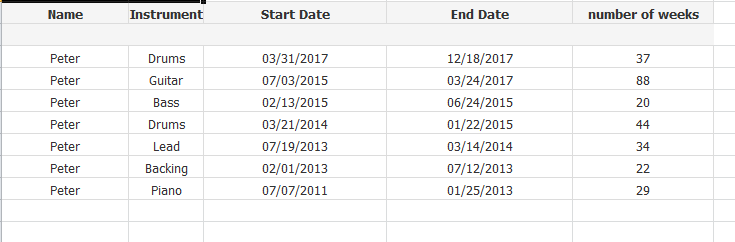# QlikView App Development

Discussion Board for collaboration related to QlikView App Development.

New Contributor

## Grouping calculation

Hi,

I'm hoping this is simple.

I want to group the Instrument with Start and End Dates, and ultimately calculate the total number of weeks attached to each.

I have attached two screenshots below. The 1st is the raw dataset, and the 2nd is what I want to achieve in Qlikview. How do I do it?? Any help would be great.

Thanks

DatasetExpected Results1 Solution

Accepted SolutionsMVP

## Re: Grouping calculation

Try this

Table:

Instrument,

[Start Date],

[End Date],

[number of weeks]

FROM

(biff, embedded labels, table is Sheet1\$);

TempTable:

LOAD If(Name = Previous(Name), If(Instrument = Previous(Instrument), Peek('Sort'), RangeSum(Peek('Sort'), 1)), 1) as Sort,

*

Resident Table

Order By Name, [Start Date] desc;

FinalTable:

NoConcatenate

Instrument,

Sort,

Min([Start Date]) as [Start Date],

Max([End Date]) as [End Date],

Sum([number of weeks]) as [number of weeks]

Resident TempTable

Group By Name, Instrument, Sort;

DROP Tables Table, TempTable;3 Replies
Esteemed Contributor III

## Re: Grouping calculation

What is the grouping logic you used here? On what criteria you considered Drums which is coming twice?MVP

## Re: Grouping calculation

Try this

Table:

Instrument,

[Start Date],

[End Date],

[number of weeks]

FROM

(biff, embedded labels, table is Sheet1\$);

TempTable:

LOAD If(Name = Previous(Name), If(Instrument = Previous(Instrument), Peek('Sort'), RangeSum(Peek('Sort'), 1)), 1) as Sort,

*

Resident Table

Order By Name, [Start Date] desc;

FinalTable:

NoConcatenate

Instrument,

Sort,

Min([Start Date]) as [Start Date],

Max([End Date]) as [End Date],

Sum([number of weeks]) as [number of weeks]

Resident TempTable

Group By Name, Instrument, Sort;

DROP Tables Table, TempTable;New Contributor

## Re: Grouping calculation

Excellent. Thank you Sunny